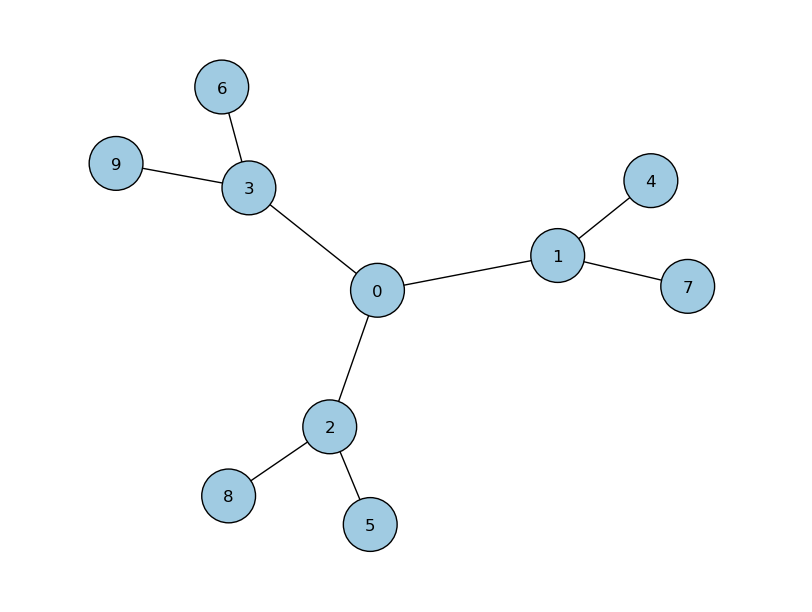# 1P_3cayley.py¶

## Description¶This example propagates a 1 particle continuous-time quantum walk on a 3-cayley tree. Amongst the features used, it illustrates: the use of the chebyshev algorithm, with eigenvalues pre-set adding a diagonal defect of amplitude 3 to node 0.This acts to alter the original Hamiltonian, by the addition of a diagonal ‘disorder’ matrix $$\Gamma$$; $$H = H_0 + \Gamma$$ where $$\Gamma = 3|0\rangle\langle 0|$$. creating node handles to watch the probability at specified nodes various plotting abilities: probability vs node plots probability vs time plots graph plots

## Source Code¶

  1 2 3 4 5 6 7 8 9 10 11 12 13 14 15 16 17 18 19 20 21 22 23 24 25 26 27 28 29 30 31 32 33 34 35 36 37 38 39 40 41 42 43 44 45 46 47 48 49 50 51 52 53 54 55 56 57 #!/usr/bin/env python2.7 # initialize PETSc import sys, petsc4py petsc4py.init(sys.argv) from petsc4py import PETSc import numpy as np # import pyCTQW as qw import pyCTQW.MPI as qw # enable command line arguments -t and -N OptDB = PETSc.Options() N = OptDB.getInt('N', 10) t = OptDB.getReal('t', 20) # get the MPI rank rank = PETSc.Comm.Get_rank(PETSc.COMM_WORLD) if rank == 0: print '1P 3-cayley Tree CTQW\n' # initialise a 10 node graph CTQW walk = qw.Graph(N) # Create a Hamiltonian from a file. # A defect of amplitude 3 has been added to node 0 walk.createH('../graphs/cayley/3-cayley.txt','txt',d=,amp=[3.]) # create the initial state (1/sqrt(2)) (|0>+i|1>) init_state = [[0,1.0/np.sqrt(2.0)], [1,1.0j/np.sqrt(2.0)]] walk.createInitState(init_state) # set the eigensolver properties. Note that # Emin is exact, whereas and Emax has been over-estimated. walk.EigSolver.setEigSolver(tol=1.e-2,verbose=False,emin_estimate=0.,emax_estimate=10.) # create a handle to watch the probability at nodes 0-4: walk.watch([0,1,2,3,4]) # Propagate the CTQW using the Chebyshev method # for t=20s in timesteps of dt=0.01 for dt in np.arange(0.01,t+0.01,0.01): walk.propagate(dt,method='chebyshev') # plot the marginal probabilities # after propagation over all nodes walk.plot('out/1p_3cayley_plot.png') # plot a 3D graph representation with bars # representing the marginal probabilities walk.plotGraph(output='out/1p_3cayley_graph.png') # plot the probability over time for the watched nodes walk.plotNodes('out/1p_3cayley_nodes.png') # destroy the quantum walk walk.destroy()